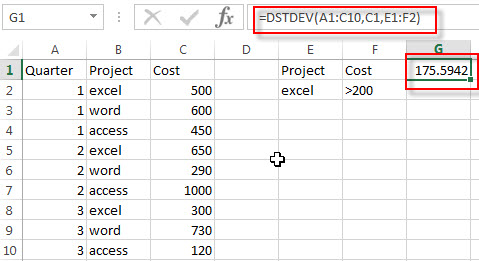# Excel DSTDEV Function

This post will guide you how to use Excel DSTDEV function with syntax and examples in Microsoft excel.

### Description

The Excel DSTDEV returns the standard deviation of a population based on a sample of numbers in a column or database that match the given criteria.

The DSTDEV function is a build-in function in Microsoft Excel and it is categorized as a Database Function.
The DSTDEV function is available in Excel 2016, Excel 2013, Excel 2010, Excel 2007, Excel 2003, Excel XP, Excel 2000, Excel 2011 for Mac.

### Syntax

The syntax of the DSTDEV function is as below:

`= DSTDEV (database, field, criteria)`

Where the DSTDEV function arguments are:

• Database -This is a required argument. The range of cells that containing the database.
• Field – This is a required argument. The column with database that you want the minimum of.
• Criteria – The range of cells that contains the conditions that you specify.

### Excel DSTDEV Function Examples

The below examples will show you how to use Excel DSTDEV Function to calculate the sample standard deviation of a column in a database or list that matching the given criteria you specify.

#1 =DSTDEV(A1:C10,C1,E1:F2)### Related Functions

• Excel DSTDEVP Function
The Excel DSTDEVP returns the standard deviation of a population based on the entire population of numbers in a column or database that match the given criteria.The syntax of the DSTDEVP function is as below:=DSTDEVP(database, field, criteria)…
Related Posts

Excel DVARP Function

This post will guide you how to use Excel DVARP function with syntax and examples in Microsoft excel. Description The Excel DVARP Function will get the variance of a population based on the entire population of numbers in a column ...

Excel DVAR Function

This post will guide you how to use Excel DVAR function with syntax and examples in Microsoft excel. Description The Excel DVAR Function will get the variance of a population based on a sample of numbers in a column in ...

Excel DSUM Function

This post will guide you how to use Excel DSUM function with syntax and examples in Microsoft excel. Description The Excel DSUM Function will add the numbers in a column or database that meets a given criteria. And so you ...

Excel DSTDEVP Function

This post will guide you how to use Excel DSTDEVP function with syntax and examples in Microsoft excel. Description The Excel DSTDEVP returns the standard deviation of a population based on the entire population of numbers in a column or ...

Excel DMIN Function

This post will guide you how to use Excel DMIN function with syntax and examples in Microsoft excel. Description The Excel DMIN returns the minimum value from a database or a column of a list that matches the specified conditions. ...

Sidebar